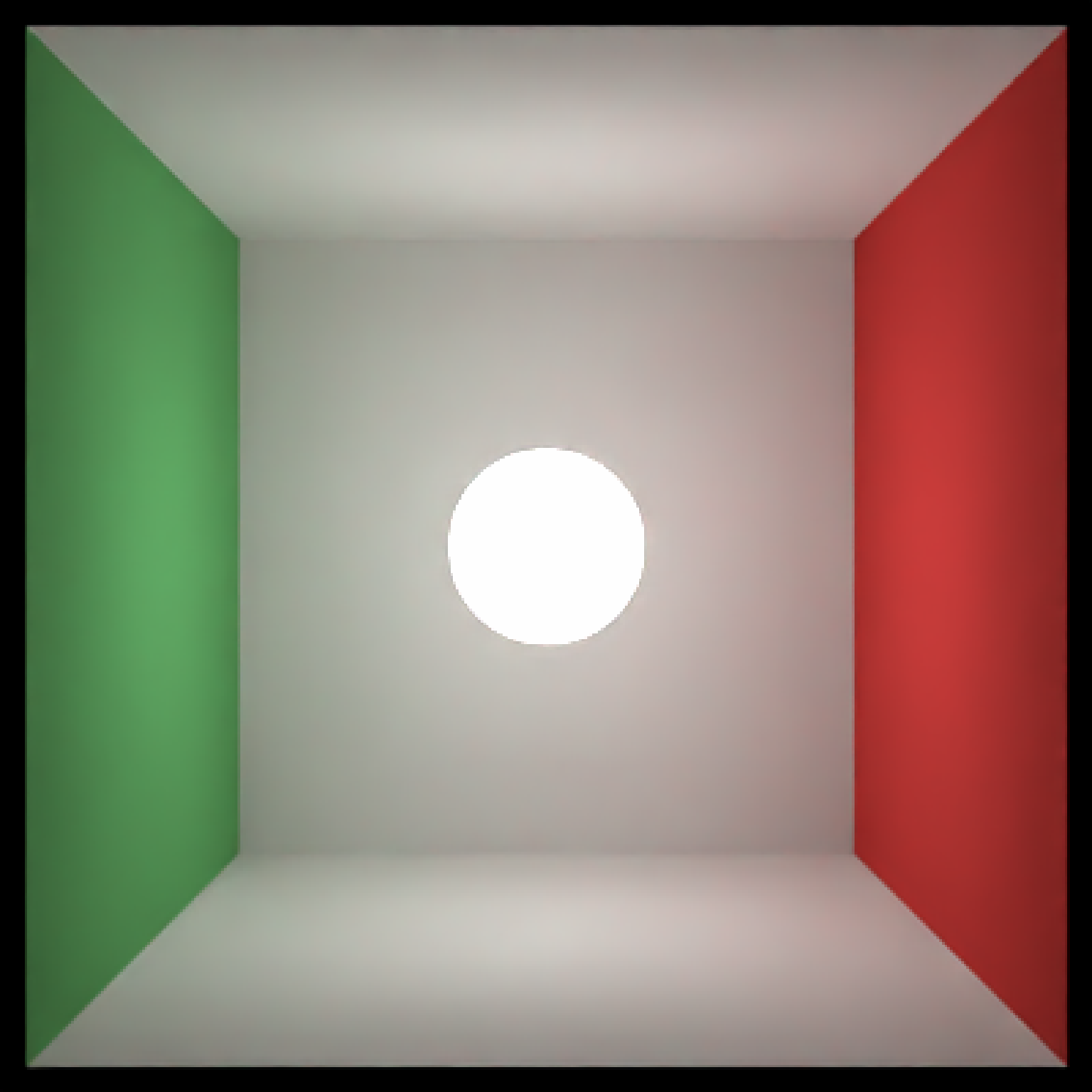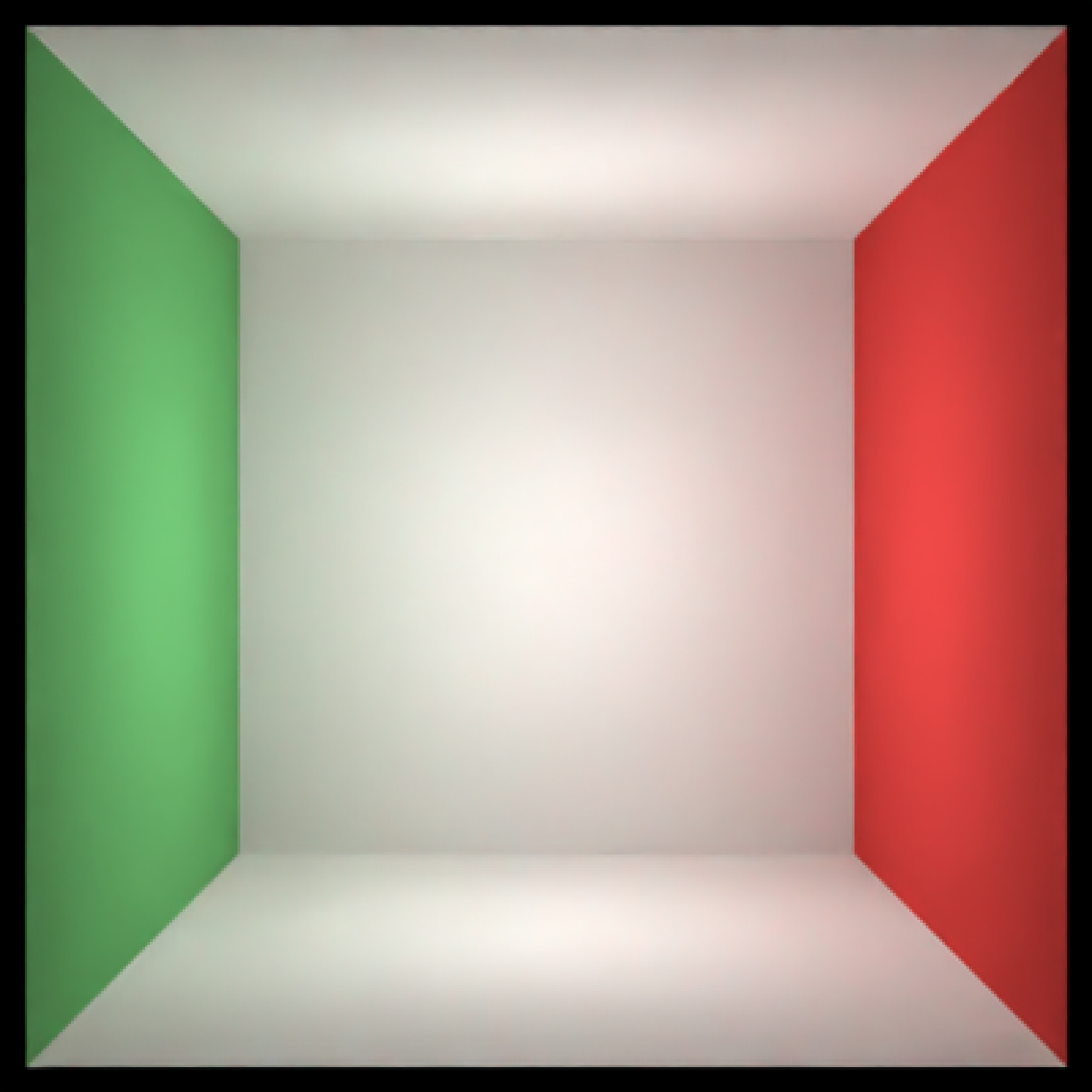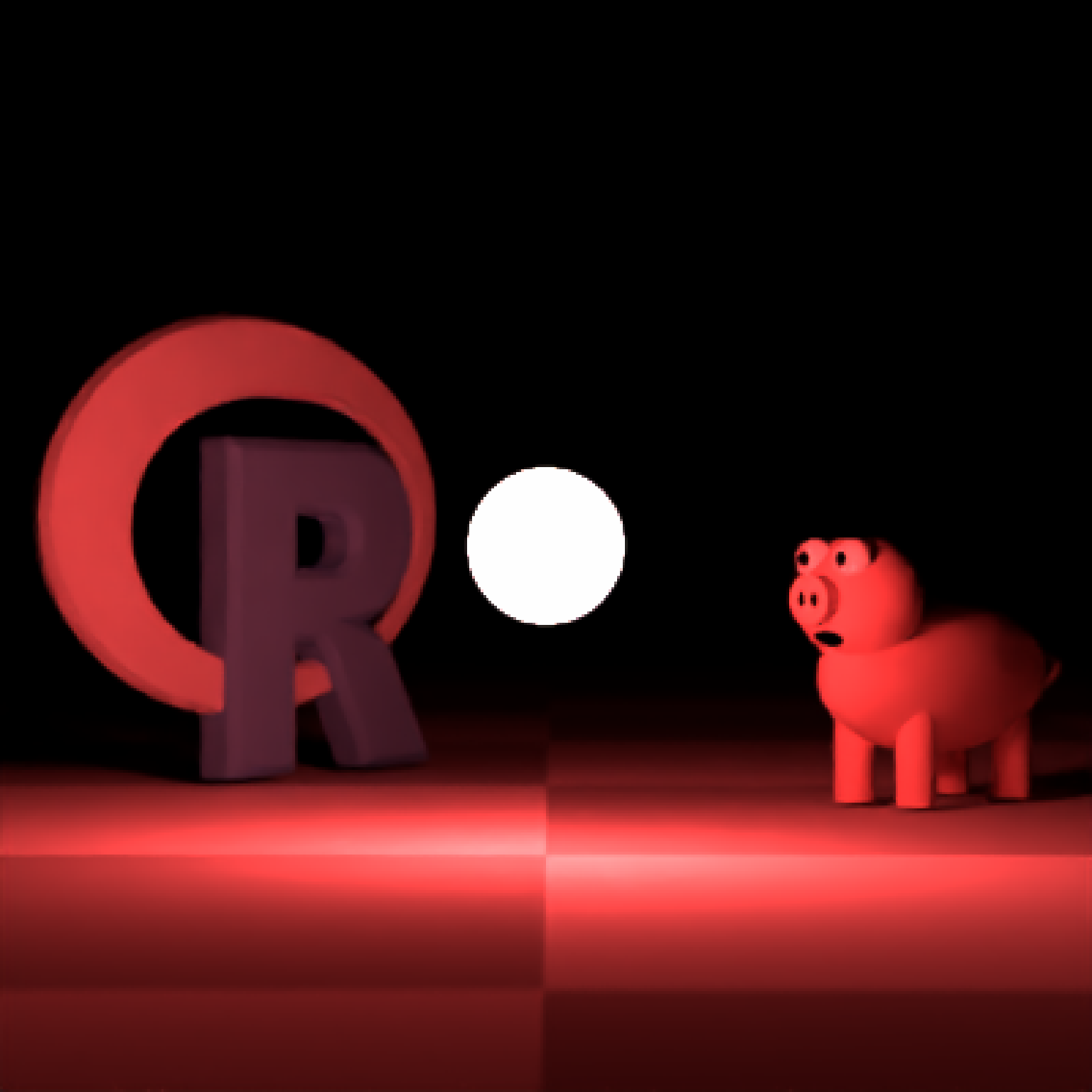Light Material

light(
color = "#ffffff",
intensity = 10,
importance_sample = TRUE,
spotlight_focus = NA,
spotlight_width = 30,
spotlight_start_falloff = 15,
invisible = FALSE,
image_texture = NA,
image_repeat = 1,
)

## Arguments

color

Default white. The color of the light Can be either a hexadecimal code, R color string, or a numeric rgb vector listing three intensities between 0 and 1.

intensity

Default 10. If a positive value, this will turn this object into a light emitting the value specified in color (ignoring other properties). Higher values will produce a brighter light.

importance_sample

Default TRUE. Keeping this on for lights improves the convergence of the rendering algorithm, in most cases. If the object is particularly important in contributing to the light paths in the image (e.g. light sources, refracting glass ball with caustics, metal objects concentrating light), this will help with the convergence of the image.

spotlight_focus

Default NA, no spotlight. Otherwise, a length-3 numeric vector specifying the x/y/z coordinates that the spotlight should be focused on. Only works for spheres and rectangles.

spotlight_width

Default 30. Angular width of the spotlight.

spotlight_start_falloff

Default 15. Angle at which the light starts fading in intensity.

invisible

Default FALSE. If TRUE, the light itself will be invisible.

image_texture

Default NA. A 3-layer RGB array or filename to be used as the texture on the surface of the object.

image_repeat

Default 1. Number of times to repeat the image across the surface. u and v repeat amount can be set independently if user passes in a length-2 vector.

Default NA. If not NA, creates a secondary color for a linear gradient between the this color and color specified in color. Direction is determined by gradient_transpose.

Default FALSE. If TRUE, this will use the v coordinate texture instead of the u coordinate texture to map the gradient.

Default NA. If not NA, this changes the behavior from mapping texture coordinates to mapping to world space coordinates. This should be a length-3 vector specifying the x,y, and z points where the gradient begins with value color.

Default NA. If not NA, this changes the behavior from mapping texture coordinates to mapping to world space coordinates. This should be a length-3 vector specifying the x,y, and z points where the gradient begins with value gradient_color.

Default hsv. Colorspace to calculate the gradient. Alternative rgb.

## Value

Single row of a tibble describing the light material.

## Examples

#Generate the cornell box without a light and add a single white sphere to the center
scene = generate_cornell(light=FALSE) %>%
if(rayrender:::run_documentation()) {
render_scene(scene, lookfrom=c(278,278,-800),lookat = c(278,278,0), samples=128,
aperture=0, fov=40, ambient_light=FALSE, parallel=TRUE)
}#Remove the light for direct camera rays, but keep the lighting
scene = generate_cornell(light=FALSE) %>%
material=light(intensity=15,invisible=TRUE)))
if(rayrender:::run_documentation()) {
render_scene(scene, lookfrom=c(278,278,-800),lookat = c(278,278,0), samples=128,
aperture=0, fov=40, ambient_light=FALSE, parallel=TRUE)
}#All gather around the orb
scene = generate_ground(material = diffuse(checkercolor="grey50")) %>%